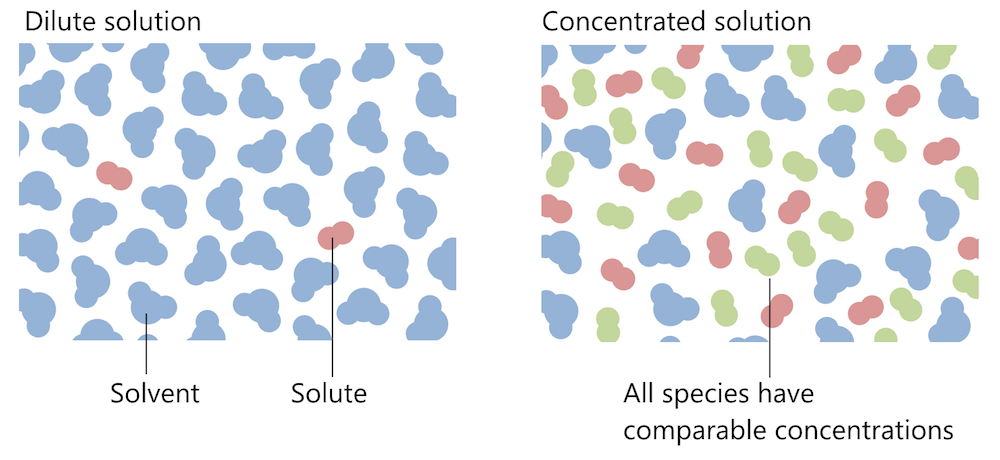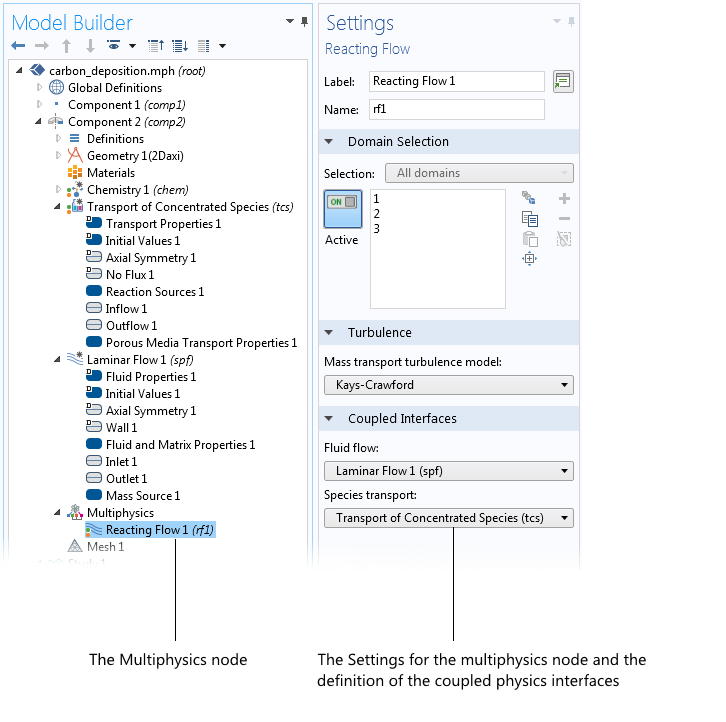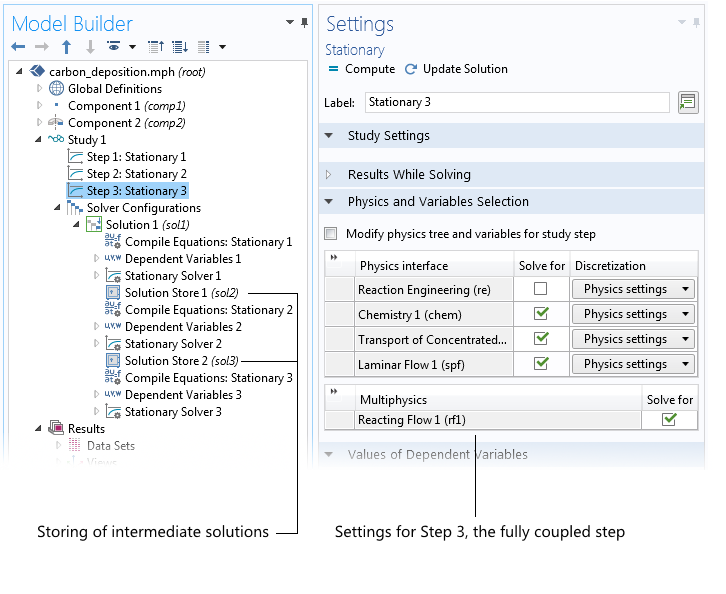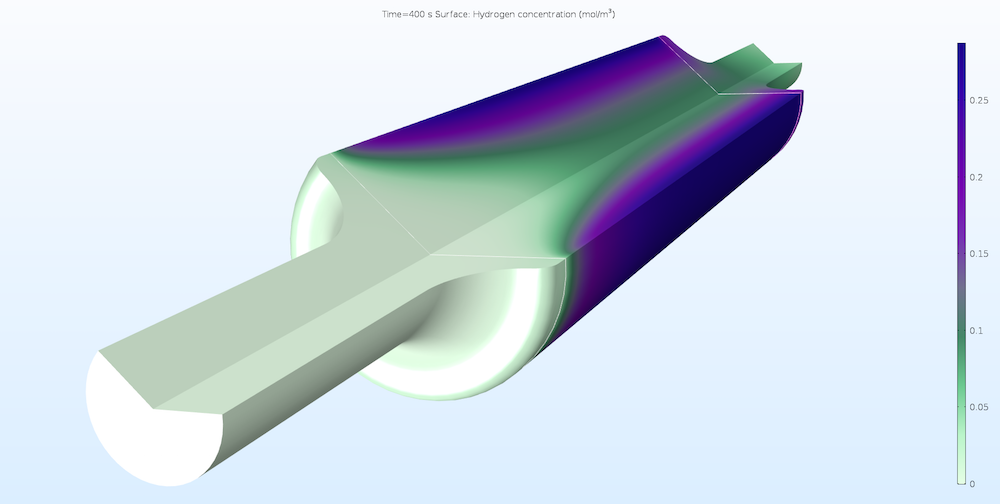## New Reacting Flow Multiphysics Interface Delivers Greater Flexibility

#####Ed Fontes September 9, 2016

In recent versions of the COMSOL Multiphysics® software, we’ve added several new multiphysics interfaces that include the constituent interfaces as separate physics interfaces, with the couplings predefined in the model tree’s Multiphysics node. This provides you with the best of both worlds, combining the flexibility of the constituent physics interfaces and the user-friendly nature of the predefined multiphysics couplings. The latest version of COMSOL Multiphysics® — version 5.2a — is no exception with the new Reacting Flow multiphysics interface.

### Studying Fluid Flow and Reactions in Gases and Liquids

The Chemical Reaction Engineering, CFD, and Plasma modules all include different variations of the equations for the transport of chemical species in a concentrated solution, such as the Maxwell-Stefan equations and the mixture-average model. In a concentrated solution, the model equations have to account for the interactions between all species in a solution, while a model of a dilute solution only includes the interaction between the solute and the solvent. The schematics below illustrates the difference between these two descriptions.Dilute solutions (left) and concentrated solutions (right). The interaction within dilute solutions is dominated by the interactions between solvent and solute and solvent with solvent. In a concentrated solution, all species interact with one another.

Along with these interactions, the velocity field in a concentrated solution is defined as the sum of the flux over every species, i:

(1)

${\mathbf{v}} = \frac{{\sum\limits_i {{{\mathbf{n}}_i}} }}{{\sum\limits_i {{\rho _i}} }}$

where n denotes the flux in kg/(m2s), and ρ represents the density (kg/m3). For a dilute solution, the velocity field is given by the velocity of the solvent:

(2)

${\mathbf{v}} \approx \frac{{{{\mathbf{n}}_{solvent}}}}{{{\rho _{solvent}}}}$

As we can see from the images above and Eq. (1), the transport of species and fluid flow are tightly coupled for concentrated solutions.

### Utilizing the New Reacting Flow Multiphysics Interface in COMSOL Multiphysics®

In earlier versions of COMSOL Multiphysics, the Reacting Flow interface was a single multiphysics interface with its own domain settings and boundary conditions, specifically designed for coupling flow and chemical species transport and reactions. This approach was user-friendly in nature, as everything was predefined. However, some of the general flexibility in COMSOL Multiphysics was lost in this predefined physics interface. Say you wanted to make larger changes separately to the transport of concentrated species equations and the flow equations. To do so, you would have to define the problem by adding the two types of physics interfaces separately, instead of using the predefined multiphysics interface, and then manually construct the multiphysics coupling.

With the new Reacting Flow interface for a concentrated solution, you can handle this tight coupling while maintaining the ability to manipulate the transport equations and the fluid flow settings separately. The coupling itself is defined in the Multiphysics node. With such functionality, you can, for instance, change from laminar flow to turbulent flow or change the transport model from the Maxwell-Stefan equations to the mixture-average model.

Let’s see how this is manifested in the model tree and in the settings for the Multiphysics node. As the following screenshot shows, all of the usual nodes for the constituent physics interfaces can be modified while the coupling is predefined in the Multiphysics node. The predefined coupling controls the mass fluxes and, when summed over all species, satisfies the continuity equation for the flow. As such, the two sets of equations are fully coupled in a bidirectional way.The model tree with the Reacting Flow multiphysics node selected. Here, we can select which physics interfaces to couple. We can also make changes to the flow model that allow us to include turbulent reacting flow. This is an additional flexibility, with preserved ease of use, as compared to previous multiphysics interfaces.

Another benefit of the new Reacting Flow multiphysics interface is found in the Study node. We have the ability to solve for the fluid flow equations to obtain a decent initial guess for the total flux. In a second step, we can solve only for the transport of species, with the velocity field given by the previous solution of the fluid flow equations.

Now we have a decent solution for the fluid flow and for the composition of the solution, which we can use as an initial guess for the fully coupled problem. Therefore, the last study step (Step 3) involves solving for the fluid flow and the transport of chemical species in a fully coupled scheme. Note though that the fully coupled scheme itself may also be sequential for a large number of species in 3D, but the loop over all species and the fluid flow is performed automatically.The three study steps (1, 2, and 3) solve for the fluid flow, the transport of chemical species, and the fully coupled problem, respectively. The automatically generated solver configuration shows the intermediate steps that store the flow field, the concentration field, and the final step that then solves for the fully coupled problem using the stored solutions as an initial guess.

By utilizing the new Reacting Flow multiphysics interface, you have the ability to solve a range of interesting problems, such as the one shown below. In this case, we can see the flow and concentration in a tubular reactor that converts methane into hydrogen. The model combines the transport of species in concentrated solutions, fluid flow in free and porous media, and heat transfer with the endothermic reactions and the heated jacket on the reactor’s cylindrical outer walls.Concentration of hydrogen in a reactor that converts methane into hydrogen. The reaction is endothermic and heat is supplied at the cylindrical walls, which yields a higher hydrogen production close to the walls.

### Learn More About Modeling Fluid Flow and Reactions in Gases and Liquids

• Download related tutorials from our Application Gallery: